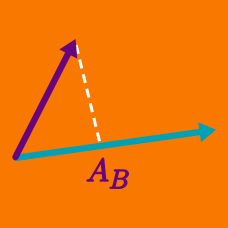Geometry

# Projecting a vector onto another vector

If the two vectors $\vec{a}$ and $\vec{b}$ are given by $\vec{a} = 4i + 3j$ and $\vec{b} = -3i + j,$ what is the difference between the length of the projection of $\vec{b}$ onto $\vec{a}$ and the length of the projection of $\vec{a}$ onto $\vec{b}?$

A line $l$ passes through the point $P(0, 4).$ Let $A$ and $B$ be the two intersection points of $l$ and the circle $x^2 + y^2= 64 .$ If $O$ is the origin and $Q$ is the intersection point of the two tangent lines at points $A$ and $B,$ what is the result of the dot product $\overrightarrow{OP} \cdot \overrightarrow{OQ}?$

Project $\vec{ u}$ onto $\vec{v},$ both defined below, and write the resulting vector in the same form as $\vec{v}:$ $\vec{u} = i - 2j \text{ and } \vec{v} = -10 i.$

Calculate the vector projection of $\vec{a} = (-2, 3)$ onto $\vec{b} = (6, 8).$

If the two vectors $\vec{a}$ and $\vec{b}$ are given by $\vec{a} = 3i + 4j$ and $\vec{b} = -2i + 4j,$ what is the difference between the length of the projection of $\vec{b}$ onto $\vec{a}$ and the length of the projection of $\vec{a}$ onto $\vec{b}?$

×